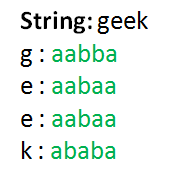Open in App
Not now

# Baconian Cipher

• Difficulty Level : Easy
• Last Updated : 13 Jul, 2022

Bacon’s cipher or the Baconian cipher is a method of steganography (a method of hiding a secret message as opposed to just a cipher) devised by Francis Bacon in 1605. A message is concealed in the presentation of text, rather than its content. The Baconian cipher is a substitution cipher in which each letter is replaced by a sequence of 5 characters. In the original cipher, these were sequences of ‘A’s and ‘B’s e.g. the letter ‘D’ was replaced by ‘aaabb’, the letter ‘O’ was replaced by ‘abbab’ etc.

Each letter is assigned to a string of five binary digits. These could be the letters ‘A’ and ‘B’, the numbers 0 and 1 or whatever else you may desire.

There are 2 kinds of Baconian ciphers –

1. The 24 letter cipher: In which 2 pairs of letters (I, J) & (U, V) have same ciphertexts.
1. The 26 letter cipher: In which all letters have unique ciphertexts.

Encryption

We will extract a single character from the string and if its not a space then we will replace it with its corresponding ciphertext according to the cipher we are using else we will add a space and repeat it until we reach the end of the string. For example ‘A’ is replaced with ‘aaaaa’Decryption

We will extract every set of 5 characters from the encrypted string and check if the first character in that set of 5 characters is a space. If not we will lookup its corresponding plaintext letter from the cipher, replace it and increment the index of character by 5 (to get the set of next 5 characters) else if its a space we add a space and repeat a process by incrementing the current index of character by 1

Approach

In Python, we can map key-value pairs using a data structure called a dictionary. We are going to use just one dictionary in which we will map the plaintext-ciphertext pairs as key-value pairs. For encryption we will simply lookup the corresponding ciphertext by accessing the value using the corresponding plaintext character as key. In decryption we will extract every 5 set of ciphertext characters and retrieve their keys from the dictionary using them as the corresponding value. For an accurate decryption we will use the 26 letter cipher. If you are not coding in python then you can come up with your own approach.

Implementation:

## Python

 `# Python program to implement Baconian cipher` `'''This script uses a dictionary instead of 'chr()' & 'ord()' function'''` `'''``Dictionary to map plaintext with ciphertext``(key:value) => (plaintext:ciphertext)``This script uses the 26 letter baconian cipher``in which I, J & U, V have distinct patterns``'''``lookup ``=` `{``'A'``: ``'aaaaa'``, ``'B'``: ``'aaaab'``, ``'C'``: ``'aaaba'``, ``'D'``: ``'aaabb'``, ``'E'``: ``'aabaa'``,``          ``'F'``: ``'aabab'``, ``'G'``: ``'aabba'``, ``'H'``: ``'aabbb'``, ``'I'``: ``'abaaa'``, ``'J'``: ``'abaab'``,``          ``'K'``: ``'ababa'``, ``'L'``: ``'ababb'``, ``'M'``: ``'abbaa'``, ``'N'``: ``'abbab'``, ``'O'``: ``'abbba'``,``          ``'P'``: ``'abbbb'``, ``'Q'``: ``'baaaa'``, ``'R'``: ``'baaab'``, ``'S'``: ``'baaba'``, ``'T'``: ``'baabb'``,``          ``'U'``: ``'babaa'``, ``'V'``: ``'babab'``, ``'W'``: ``'babba'``, ``'X'``: ``'babbb'``, ``'Y'``: ``'bbaaa'``, ``'Z'``: ``'bbaab'``}` `# Function to encrypt the string according to the cipher provided`  `def` `encrypt(message):``    ``cipher ``=` `''``    ``for` `letter ``in` `message:``        ``# checks for space``        ``if``(letter !``=` `' '``):``            ``# adds the ciphertext corresponding to the``            ``# plaintext from the dictionary``            ``cipher ``+``=` `lookup[letter]``        ``else``:``            ``# adds space``            ``cipher ``+``=` `' '` `    ``return` `cipher` `# Function to decrypt the string``# according to the cipher provided`  `def` `decrypt(message):``    ``decipher ``=` `''``    ``i ``=` `0` `    ``# emulating a do-while loop``    ``while` `True``:``        ``# condition to run decryption till``        ``# the last set of ciphertext``        ``if``(i < ``len``(message)``-``4``):``            ``# extracting a set of ciphertext``            ``# from the message``            ``substr ``=` `message[i:i ``+` `5``]``            ``# checking for space as the first``            ``# character of the substring``            ``if``(substr[``0``] !``=` `' '``):``                ``'''``                ``This statement gets us the key(plaintext) using the values(ciphertext)``                ``Just the reverse of what we were doing in encrypt function``                ``'''``                ``decipher ``+``=` `list``(lookup.keys()``                                 ``)[``list``(lookup.values()).index(substr)]``                ``i ``+``=` `5`  `# to get the next set of ciphertext` `            ``else``:``                ``# adds space``                ``decipher ``+``=` `' '``                ``i ``+``=` `1`  `# index next to the space``        ``else``:``            ``break`  `# emulating a do-while loop` `    ``return` `decipher`  `def` `main():``    ``message ``=` `"Geeks for Geeks"``    ``result ``=` `encrypt(message.upper())``    ``print``(result)` `    ``message ``=` `"AABAAABBABABAABABBBABBAAA"``    ``result ``=` `decrypt(message.lower())``    ``print``(result)`  `# Executes the main function``if` `__name__ ``=``=` `'__main__'``:``    ``main()`

Output

```aabbaaabaaaabaaabababaaba aabababbbabaaab aabbaaabaaaabaaabababaaba
ENJOY```

Analysis: This cipher offers very little communication security, as it is a substitution cipher. As such all the methods used to cryptanalyse substitution ciphers can be used to break Baconian ciphers. The main advantage of the cipher is that it allows hiding the fact that a secret message has been sent at all.

This article is contributed by Palash Nigam . If you like GeeksforGeeks and would like to contribute, you can also write an article using write.geeksforgeeks.org or mail your article to review-team@geeksforgeeks.org. See your article appearing on the GeeksforGeeks main page and help other Geeks.

My Personal Notes arrow_drop_up